/

### Function Description

Returns the weighted (sample) covariance between two series.

Weighted (sample) covariances, given weights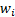(for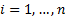), are calculated as: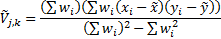where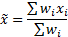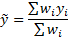See Weighted Moments and Cumulants for further details and explanation of terms in this formula. If the weights are equal then returns the same as MnCovariance.

Contents | Prev | Next